# Merging deep learning with physical models

## François Lanusse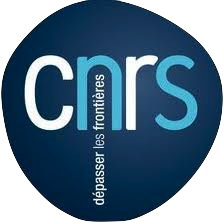slides at eiffl.github.io/talks/IAIFI2023

### the Rubin Observatory Legacy Survey of Space and Time

• 1000 images each night, 15 TB/night for 10 years

• 18,000 square degrees, observed once every few days

• Tens of billions of objects, each one observed $\sim1000$ times

Previous generation survey: SDSS

Image credit: Peter Melchior

Current generation survey: DES

Image credit: Peter Melchior

LSST precursor survey: HSC

Image credit: Peter Melchior

### We need to rethink all stages of data analysis for modern surveys

Bosch et al. 2017

Jeffrey, Lanusse, et al. 2020

Cheng et al. 2020
• Galaxies are no longer blobs.
• Signals are no longer Gaussian.
• Cosmological likelihoods are no longer tractable.

$\Longrightarrow$ This is the end of the analytic era...

### ... but the beginning of the data-driven era

Case I: Examples from data, no accurate physical model

Mandelbaum et al. 2014

Case II: Physical model only available as a simulator

Osato et al. 2020

$\Longrightarrow$ Examples of implicit distributions: we have access to samples $\{x_0, x_1, \ldots, x_n \}$ but we cannot evaluate $p(x)$.

## How can we leverage implicit distributions for physical Bayesian inference?

### The answer is: Deep Generative Modeling

• The goal of generative modeling is to learn an implicit distribution $\mathbb{P}$ from which the training set $X = \{x_0, x_1, \ldots, x_n \}$ is drawn.

• Usually, this means building a parametric model $\mathbb{P}_\theta$ that tries to be close to $\mathbb{P}$.

True $\mathbb{P}$

Samples $x_i \sim \mathbb{P}$

Model $\mathbb{P}_\theta$

• Once trained, you can typically sample from $\mathbb{P}_\theta$ and/or evaluate the likelihood $p_\theta(x)$.

### Why isn't it easy?

• The curse of dimensionality put all points far apart in high dimension

Distance between pairs of points drawn from a Gaussian distribution.

• Classical methods for estimating probability densities, i.e. Kernel Density Estimation (KDE) start to fail in high dimension because of all the gaps

### The evolution of generative models

• Deep Belief Network
(Hinton et al. 2006)

• Variational AutoEncoder
(Kingma & Welling 2014)

(Goodfellow et al. 2014)

• Wasserstein GAN
(Arjovsky et al. 2017)

• Midjourney v5 Guided Latent Diffusion (2023)

$\Longrightarrow$ We can consider the problem of learning distributions to be essentially solved even in high dimensions.

### Focus of this talk

This talk
Generic approach to uncertainty quantification and interpretability:
• (Differentiable) Physical Forward Models
• Deep Generative Models
• Bayesian Inference

# Solving Inverse Problems

## with deep implicit priors

Branched GAN model for deblending (Reiman & Göhre, 2018)
The issue with using deep learning as a black-box
• No explicit control of noise, PSF, number of sources.
• Model would have to be retrained for all observing configurations

• No guarantees on the network output (e.g. flux preservation, artifacts)

• No proper uncertainty quantification.

### Linear inverse problems

$\boxed{y = \mathbf{A}x + n}$

$\mathbf{A}$ is known and encodes our physical understanding of the problem.
$\Longrightarrow$ When non-invertible or ill-conditioned, the inverse problem is ill-posed with no unique solution $x$
Deconvolution
Inpainting
Denoising

### What Would a Bayesian Do?

$\boxed{y = \mathbf{A}x + n}$

The Bayesian view of the problem:
$$p(x | y) \propto p(y | x) \ p(x)$$
• $p(y | x)$ is the data likelihood, which contains the physics

• $p(x)$ is the prior knowledge on the solution.

With these concepts in hand we can:
• Estimate for instance the Maximum A Posteriori solution:
$$\hat{x} = \arg\max\limits_x \ \log p(y \ | \ x) + \log p(x)$$
• Estimate from the full posterior p(x|y) with MCMC or Variational Inference methods.

### Classical examples of signal priors

Sparse
$$\log p(x) = \parallel \mathbf{W} x \parallel_1$$
Gaussian $$\log p(x) = x^t \mathbf{\Sigma^{-1}} x$$
Total Variation $$\log p(x) = \parallel \nabla x \parallel_1$$

### Getting started with Deep Priors: deep denoising example

$$\boxed{{\color{Orchid} y} = {\color{SkyBlue} x} + n}$$
• Let us assume we have access to examples of ${\color{SkyBlue} x}$ without noise.

• We learn the distribution of noiseless data $\log p_\theta(x)$ from samples using a deep generative model.

• The solution should lie on the realistic data manifold, symbolized by the two-moons distribution.

We want to solve for the Maximum A Posterior solution:

$$\arg \max - \frac{1}{2} \parallel {\color{Orchid} y} - {\color{SkyBlue} x} \parallel_2^2 + \log p_\theta({\color{SkyBlue} x})$$ This can be done by gradient descent as long as one has access to the score function $\frac{\color{orange} d \color{orange}\log \color{orange}p\color{orange}(\color{orange}x\color{orange})}{\color{orange} d \color{orange}x}$.

## High-Dimensional Bayesian Inference for Inverse Problems With Neural Score Estimation### Work in collaboration with: Benjamin Remy, Zaccharie Ramzi

$\Longrightarrow$ Learn complex priors by Neural Score Estimation and sample from posterior with gradient-based MCMC.

### Let's set the stage: Gravitational lensing

Galaxy shapes as estimators for gravitational shear
$$e = \gamma + e_i \qquad \mbox{ with } \qquad e_i \sim \mathcal{N}(0, I)$$
• We are trying the measure the ellipticity $e$ of galaxies as an estimator for the gravitational shear $\gamma$

### Gravitational Lensing as an Inverse Problem

Shear $\gamma$
Convergence $\kappa$
$$\gamma_1 = \frac{1}{2} (\partial_1^2 - \partial_2^2) \ \Psi \quad;\quad \gamma_2 = \partial_1 \partial_2 \ \Psi \quad;\quad \kappa = \frac{1}{2} (\partial_1^2 + \partial_2^2) \ \Psi$$
$$\boxed{\gamma = \mathbf{P} \kappa}$$

### Illustration on the Dark Energy Survey (DES) Y3

Jeffrey, et al. (2021)

### Writing down the convergence map log posterior

$$\log p( \kappa | e) = \underbrace{\log p(e | \kappa)}_{\simeq -\frac{1}{2} \parallel e - P \kappa \parallel_\Sigma^2} + \log p(\kappa) +cst$$
• The likelihood term is known analytically, given to us by the physics of gravitational lensing.
• There is no close form expression for the prior on dark matter maps $\kappa$.
However:
• We do have access to samples of full implicit prior through simulations: $X = \{x_0, x_1, \ldots, x_n \}$ with $x_i \sim \mathbb{P}$
$\Longrightarrow$ Our strategy: Learn the prior from simulation, and then sample the full posterior.

## How do you do this in practice in very high dimensional problems?

### First realization: The score is all you need!

• Whether you are looking for the MAP or sampling with HMC or MALA, you only need access to the score of the posterior: $$\frac{\color{orange} d \color{orange}\log \color{orange}p\color{orange}(\color{orange}x \color{orange}|\color{orange} y\color{orange})}{\color{orange} d \color{orange}x}$$
• Gradient descent: $x_{t+1} = x_t + \tau \nabla_x \log p(x_t | y)$
• Langevin algorithm: $x_{t+1} = x_t + \tau \nabla_x \log p(x_t | y) + \sqrt{2\tau} n_t$

• The score of the full posterior is simply: $$\nabla_x \log p(x |y) = \underbrace{\nabla_x \log p(y |x)}_{\mbox{known explicitly}} \quad + \quad \underbrace{\nabla_x \log p(x)}_{\mbox{known implicitly}}$$ $\Longrightarrow$ "all" we have to do is model/learn the score of the prior.

### Neural Score Estimation by Denoising Score Matching (Vincent 2011)

• Denoising Score Matching: An optimal Gaussian denoiser learns the score of a given distribution.
• If $x \sim \mathbb{P}$ is corrupted by additional Gaussian noise $u \in \mathcal{N}(0, \sigma^2)$ to yield $$x^\prime = x + u$$
• Let's consider a denoiser $r_\theta$ trained under an $\ell_2$ loss: $$\mathcal{L}=\parallel x - r_\theta(x^\prime, \sigma) \parallel_2^2$$
• The optimal denoiser $r_{\theta^\star}$ verifies: $$\boxed{\boldsymbol{r}_{\theta^\star}(\boldsymbol{x}', \sigma) = \boldsymbol{x}' + \sigma^2 \nabla_{\boldsymbol{x}} \log p_{\sigma^2}(\boldsymbol{x}')}$$
$\boldsymbol{x}'$
$\boldsymbol{x}$
$\boldsymbol{x}'- \boldsymbol{r}^\star(\boldsymbol{x}', \sigma)$
$\boldsymbol{r}^\star(\boldsymbol{x}', \sigma)$

### Second Realization: Annealing is everything!

• Even with knowledge of the score, sampling in high number of dimensions is difficult!

• Convolving a target distribution $p$ with a noise kernel, makes $p_\sigma(x) = \int \mathcal{N}(x; x^\prime, \sigma^2) (x^\prime) d x^{\prime}$ it much better behaved
$$\sigma_1 > \sigma_2 > \sigma_3 > \sigma_4$$
• Hints to running many MCMC chains in parallel, progressively annealing the $\sigma$ to 0, keep last point in the chain as independent sample.

### Score-Based Generative Modeling Song et al. (2021)

• The SDE defines a marginal distribution $p_t(x)$ as the convolution of the target distribution $p(x)$ with a noise kernel $p_{t|s}(\cdot | x_s)$: $$p_t(x) = \int p(x_s) p_{t|s}(x | x_s) d x_s$$
• For a given forward SDE that evolves $p(x)$ to $p_T(x)$, there exists a reverse SDE that evolves $p_T(x)$ back into $p(x)$. It involves having access to the marginal score $\nabla_x \log_t p(x)$.

### Third realization: We don't actually know the marginal posterior score!

• We know the following quantities:
• Annealed likelihood (analytically): $p_\sigma(y | x) = \mathcal{N}(y; \mathbf{A} x, \mathbf{\Sigma} + \sigma^2 \mathbf{I})$
• Annealed prior score (by score matching): $\nabla_x \log p_\sigma(x)$
• But, unfortunately: $\boxed{p_\sigma(x|y) \neq p_\sigma(y|x) \ p_\sigma(x)}$ $\Longrightarrow$ We don't know the marginal posterior score!
• We cannot use the reverse SDE/ODE of diffusion models to sample from the posterior. $$\mathrm{d} x = [f(x, t) - g^2(t) \underbrace{\nabla_x \log p_t(x|y)}_{\mbox{unknown}} ] \mathrm{d}t + g(t) \mathrm{d} w$$
Proposed sampling strategy
• Even if not equivalent to the marginal posterior score, $\nabla_x \log p_{\sigma^2}(y | x) + \nabla_x \log p_{\sigma^2}(x)$ still has good properties:
• Tends to an isotropic Gaussian distribution for large $\sigma$
• Corresponds to the target posterior for $\sigma=0$

• If we simulate this SDE sufficiently slowly (i.e. timescale of change of $\sigma$ is much larger than the timescale of the SDE) we can expect to sample from the target posterior.
$\Longrightarrow$ In practice, we sample the annealed distribution using an Hamiltonian Monte-Carlo, with discrete annealing steps.

### Illustration on $\kappa$-TNG simulations

True convergence map
Wiener Filter
Posterior Mean (ours)

Posterior samples

### Reconstruction of the HST/ACS COSMOS field

Massey et al. (2007)
Remy et al. (2022) Posterior mean
Remy et al. (2022) Posterior samples

### Other examples of Deep Priors

$\mathcal{L} = \frac{1}{2} \parallel \mathbf{\Sigma}^{-1/2} (\ Y - P \ast A S \ ) \parallel_2^2 - \sum_{i=1}^K \log p_{\theta}(S_i)$

## Variational Inference over Hybrid Hierarchical Bayesian Models### Work led by Benjamin Remy

$\Longrightarrow$ Eliminate model bias in shear inference by using data-driven morphology priors.

### Impact of galaxy morphology on shape measurement

Mandelbaum, et al. (2013), Mandelbaum, et al. (2014)

$\Longrightarrow$ Let's learn a prior from this implicit distribution!

### Complications specific to astronomical images: spot the differences!

CelebA

HSC PDR-2 wide

• There is noise
• We have a Point Spread Function (instrumental response)

### A Physicist's approach: let's build a model

$\longrightarrow$
$g_\theta$
$\longrightarrow$
PSF
$\longrightarrow$
Pixelation
$\longrightarrow$
Noise
Probabilistic model
$$x \sim ?$$
$$x \sim \mathcal{N}(z, \Sigma) \quad z \sim ?$$
latent $z$ is a denoised galaxy image
$$x \sim \mathcal{N}( \mathbf{P} z, \Sigma) \quad z \sim ?$$
latent $z$ is a super-resolved and denoised galaxy image
$$x \sim \mathcal{N}( \mathbf{P} (\Pi \ast z), \Sigma) \quad z \sim ?$$
latent $z$ is a deconvolved, super-resolved, and denoised galaxy image
$$x \sim \mathcal{N}( \mathbf{P} (\Pi \ast g_\theta(z)), \Sigma) \quad z \sim \mathcal{N}(0, \mathbf{I})$$
latent $z$ is a Gaussian sample
$\theta$ are parameters of the model

$\Longrightarrow$ Decouples the morphology model from the observing conditions.

### How to train your dragon model

• Training the generative amounts to finding $\theta_\star$ that maximizes the marginal likelihood of the model: $$p_\theta(x | \Sigma, \Pi) = \int \mathcal{N}( \Pi \ast g_\theta(z), \Sigma) \ p(z) \ dz$$
$\Longrightarrow$ This is generally intractable

• Efficient training of parameter $\theta$ is made possible by Amortized Variational Inference.
Auto-Encoding Variational Bayes (Kingma & Welling, 2014)
• We introduce a parametric distribution $q_\phi(z | x, \Pi, \Sigma)$ which aims to model the posterior $p_{\theta}(z | x, \Pi, \Sigma)$.

• Working out the KL divergence between these two distributions leads to: $$\log p_\theta(x | \Sigma, \Pi) \quad \geq \quad - \mathbb{D}_{KL}\left( q_\phi(z | x, \Sigma, \Pi) \parallel p(z) \right) \quad + \quad \mathbb{E}_{z \sim q_{\phi}(. | x, \Sigma, \Pi)} \left[ \log p_\theta(x | z, \Sigma, \Pi) \right]$$ $\Longrightarrow$ This is the Evidence Lower-Bound, which is differentiable with respect to $\theta$ and $\phi$.

### The famous Variational Auto-Encoder

$$\log p_\theta(x| \Sigma, \Pi ) \geq - \underbrace{\mathbb{D}_{KL}\left( q_\phi(z | x, \Sigma, \Pi) \parallel p(z) \right)}_{\mbox{code regularization}} + \underbrace{\mathbb{E}_{z \sim q_{\phi}(. | x, \Sigma, \Pi)} \left[ \log p_\theta(x | z, \Sigma, \Pi) \right]}_{\mbox{reconstruction error}}$$

### Sampling from the model

Woups... what's going on?

### Tradeoff between code regularization and image quality

$$\log p_\theta(x| \Sigma, \Pi ) \geq - \underbrace{\mathbb{D}_{KL}\left( q_\phi(z | x, \Sigma, \Pi) \parallel p(z) \right)}_{\mbox{code regularization}} + \underbrace{\mathbb{E}_{z \sim q_{\phi}(. | x, \Sigma, \Pi)} \left[ \log p_\theta(x | z, \Sigma, \Pi) \right]}_{\mbox{reconstruction error}}$$

### Latent space modeling with Normalizing Flows

$\Longrightarrow$ All we need to do is sample from the aggregate posterior of the data instead of sampling from the prior.

Dinh et al. 2016
Normalizing Flows
• Assumes a bijective mapping between data space $x$ and latent space $z$ with prior $p(z)$: $$z = f_{\theta} ( x ) \qquad \mbox{and} \qquad x = f^{-1}_{\theta}(z)$$
• Admits an explicit marginal likelihood: $$\log p_\theta(x) = \log p(z) + \log \left| \frac{\partial f_\theta}{\partial x} \right|(x)$$

## How do I use my generative model to infer gravitational lensing?

### Let's again think as physicists

$\longrightarrow$
$g_\theta$
$\longrightarrow$
shear $\gamma$
$\longrightarrow$
PSF
$\longrightarrow$
Noise
Probabilistic model
$$x \sim \mathcal{N}(\Pi \ast (g_\theta(z) \otimes \gamma), \Sigma) \quad z \sim \mathcal{N}(0, \mathbf{I})$$
latent $z$ are morphological parameters
$\theta$ are global parameters of the model
$\gamma$ are shear parameters

$\Longrightarrow$ We have a hybrid probabilistic model, with the known physics of lensing and of the instrument, and learned morphology model.

### Joint inference using a parametric model for the morphology

Let's assume that $g(z)$ is a sersic model, i.e. $z = \{n, r_\text{hlr}, F, e_1, e_2, s_x, s_y\}$ and $$g(z) = F \times I_0 \exp \left( -b_n \left[\left( \frac{r}{r_\text{hlr}}\right)^{\frac{1}{n}} -1\right] \right)$$
The joint inference of $p(z, \gamma | \mathcal{D})$ leads to a biased posterior...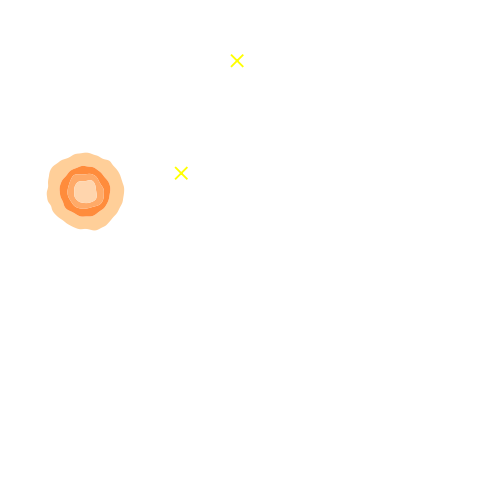Marginal shear posterior $p(\gamma|\mathcal{D})$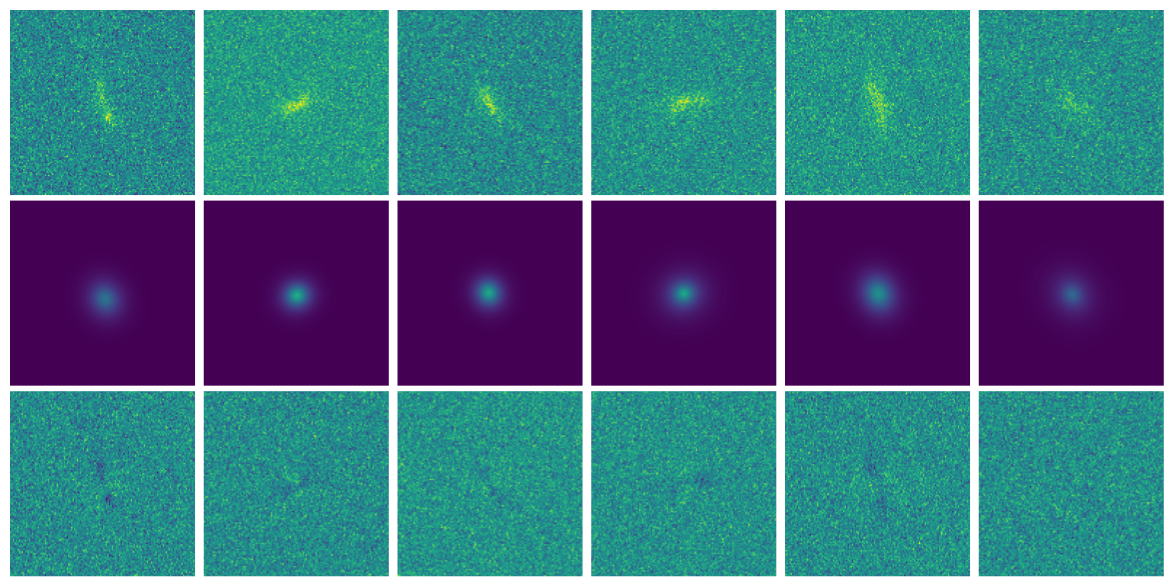Maximum a posteriori fit and residuals

We need a more realistic model of galaxy morphology

### Joint inference using a generative model for the morpholgy

Let's use a learned $g_\theta(z)$

The joint inference of $p(z, \gamma | \mathcal{D})$ leads to an unbiased posterior!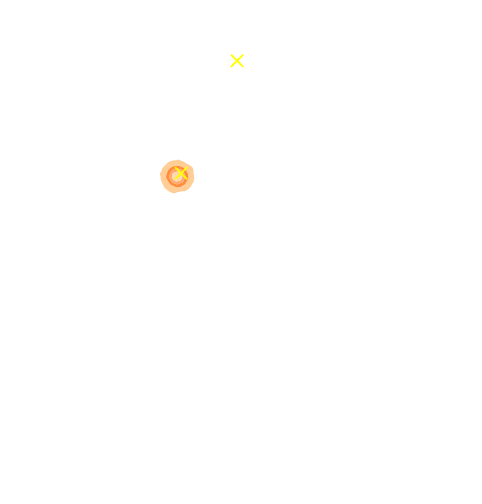Marginal shear posterior $p(\gamma|\mathcal{D})$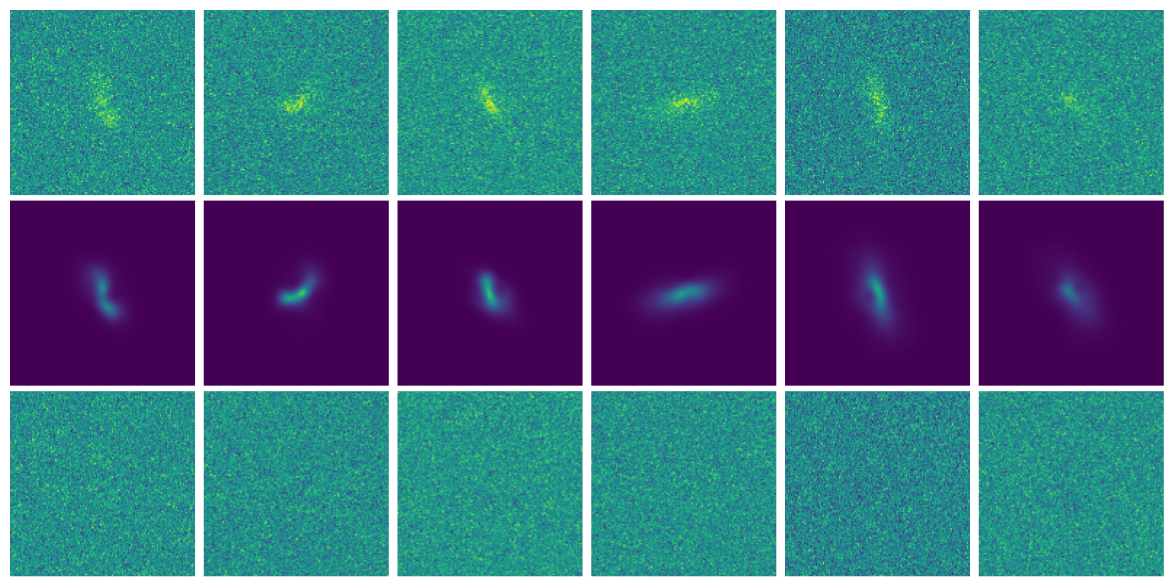Maximum a posteriori fit and residuals

### takeaways

Benefits of Bayesian forward modeling for inverse problems
Using a Bayesian approach has great advantages: model-based physical interpretation & uncertainty quantification.

• Explicit likelihood, uses of all of our physical knowledge.
$\Longrightarrow$ The method can be applied for varying noise, observing conditions, or different instruments

• Deep generative models can be used to provide simulation or data driven priors.
$\Longrightarrow$ Embed prior only accessible from samples (e.g. numerical simulations or data).

• For the first time, we have the tools to sample complex high-dimensional posteriors!

# Simulation-Based Inference

## Leveraging Physical Simulators for Inference

### The limits of traditional cosmological inference

HSC cosmic shear power spectrum
HSC Y1 constraints on $(S_8, \Omega_m)$
(Hikage et al. 2018)
• Measure the ellipticity $\epsilon = \epsilon_i + \gamma$ of all galaxies
$\Longrightarrow$ Noisy tracer of the weak lensing shear $\gamma$

• Compute summary statistics based on 2pt functions,
e.g. the power spectrum

• Run an MCMC to recover a posterior on model parameters, using an analytic likelihood $$p(\theta | x ) \propto \underbrace{p(x | \theta)}_{\mathrm{likelihood}} \ \underbrace{p(\theta)}_{\mathrm{prior}}$$
Main limitation: the need for an explicit likelihood
We can only compute from theory the likelihood for simple summary statistics and on large scales

$\Longrightarrow$ We are dismissing a significant fraction of the information!

### Full-Field Simulation-Based Inference

• Instead of trying to analytically evaluate the likelihood of sub-optimal summary statistics, let us build a forward model of the full observables.
$\Longrightarrow$ The simulator becomes the physical model.

• Each component of the model is now tractable, but at the cost of a large number of latent variables.

Benefits of a forward modeling approach
• Fully exploits the information content of the data (aka "full field inference").

• Easy to incorporate systematic effects.

• Easy to combine multiple cosmological probes by joint simulations.
(Porqueres et al. 2021)
For this talk, let's ignore the elephant in the room:
Do we have reliable enough models for the full complexity of the data?

### ...so why is this not mainstream?

The Challenge of Simulation-Based Inference
$$p(x|\theta) = \int p(x, z | \theta) dz = \int p(x | z, \theta) p(z | \theta) dz$$ Where $z$ are stochastic latent variables of the simulator.

$\Longrightarrow$ This marginal likelihood is intractable!

How to perform inference over forward simulation models?

• Implicit Inference: Treat the simulator as a black-box with only the ability to sample from the joint distribution $$(x, \theta) \sim p(x, \theta)$$ a.k.a.
• Simulation-Based Inference (SBI)
• Likelihood-free inference (LFI)
• Approximate Bayesian Computation (ABC)

• Explicit Inference: Treat the simulator as a probabilistic model and perform inference over the joint posterior $$p(\theta, z | x) \propto p(x | z, \theta) p(z, \theta) p(\theta)$$ a.k.a.
• Bayesian Hierarchical Modeling (BHM)

$\Longrightarrow$ For a given simulation model, both methods should converge to the same posterior!

# Implicit Inference

### Black-box Simulators Define Implicit Distributions

• A black-box simulator defines $p(x | \theta)$ as an implicit distribution, you can sample from it but you cannot evaluate it.
• Key Idea: Use a parametric distribution model $\mathbb{P}_\varphi$ to approximate the implicit distribution $\mathbb{P}$.

### Conditional Density Estimation with Neural Networks

• I assume a forward model of the observations: \begin{equation} p( x ) = p(x | \theta) \ p(\theta) \nonumber \end{equation} All I ask is the ability to sample from the model, to obtain $\mathcal{D} = \{x_i, \theta_i \}_{i\in \mathbb{N}}$

• I am going to assume $q_\phi(\theta | x)$ a parametric conditional density

• Optimize the parameters $\phi$ of $q_{\phi}$ according to \begin{equation} \min\limits_{\phi} \sum\limits_{i} - \log q_{\phi}(\theta_i | x_i) \nonumber \end{equation} In the limit of large number of samples and sufficient flexibility \begin{equation} \boxed{q_{\phi^\ast}(\theta | x) \approx p(\theta | x)} \nonumber \end{equation}
$\Longrightarrow$ One can asymptotically recover the posterior by optimizing a parametric estimator over
the Bayesian joint distribution
$\Longrightarrow$ One can asymptotically recover the posterior by optimizing a Deep Neural Network over
a simulated training set.

### My Practical Recipe to Apply Neural Density Estimation

A two-steps approach to Likelihood-Free Inference
• Step I Automatically learn a optimal low-dimensional summary statistic $$y = f_\varphi(x)$$ typically $y$ will have the same dimensionality as $\theta$.

• Step II: Use Neural Density Estimation in low dimension to either:
• build an estimate $p_\phi$ of the likelihood function $p(y \ | \ \theta)$ (Neural Likelihood Estimation)

• build an estimate $p_\phi$ of the posterior distribution $p(\theta \ | \ y)$ (Neural Posterior Estimation)

### Automated Summary Statistics Extraction

• Introduce a parametric function $f_\varphi$ to reduce the dimensionality of the data while preserving information.
Information-based loss functions

### Example of application: Likelihood-Free parameter inference with DES SV

Suite of N-body + raytracing simulations: $\mathcal{D}$

## Towards Optimal Full-Field Implicit Inference by Neural Summarisation and Density Estimation### An easy-to-use validation testbed: log-normal lensing simulations

DifferentiableUniverseInitiative/sbi_lens
JAX-based log-normal lensing simulation package
• 10x10 deg$^2$ maps at LSST Y10 quality, conditioning the log-normal shift parameter on $(\Omega_m, \sigma_8, w_0)$

• Infer full-field posterior on cosmology:
• explicitly using an Hamiltonian-Monte Carlo (NUTS) sampler
• implicitly using a learned summary statistics and conditional density estimation.

### but explicit inference yields intermediate data products

simulated observed data

posterior samples $\kappa = f(z,\theta)$ with $z \sim p(z, \theta | x)$

### and not all neural compression techniques are equivalent

• Most papers applying neural techniques for inference have used sub-optimal compression techniques, e.g. Mean Square Error $$\mathcal{L} = || f_\varphi(x) - \theta ||_2^2$$ $\Longrightarrow$ This is ok, contours will simply be larger than they could be.

• A lot of papers are still relying on assuming a proxy Gaussian likelihoods, i.e. estimating a mean and covariance from simulations.
$\Longrightarrow$ This is dangerous, can lead to biased contours.

# Explicit Inference

## Automatically Differentiable Physics

### Simulators as Hierarchical Bayesian Models

• If we have access to all latent variables $z$ of the simulator, then the joint log likelihood $p(x | z, \theta)$ is explicit.

• We need to infer the joint posterior $p(\theta, z | x)$ before marginalization to yield $p(\theta | x) = \int p(\theta, z | x) dz$.
$\Longrightarrow$ Extremely difficult problem as $z$ is typically very high-dimensional.

• Necessitates inference strategies with access to gradients of the likelihood. $$\frac{d \log p(x | z, \theta)}{d \theta} \quad ; \quad \frac{d \log p(x | z, \theta)}{d z}$$ For instance: Maximum A Posterior estimation, Hamiltonian Monte-Carlo, Variational Inference.

$\Longrightarrow$ The only hope for explicit cosmological inference is to have fully-differentiable cosmological simulations!

### the hammer behind the Deep Learning revolution: Automatic Differentation

• Automatic differentiation allows you to compute analytic derivatives of arbitraty expressions:
If I form the expression $y = a * x + b$, it is separated in fundamental ops: $$y = u + b \qquad u = a * x$$ then gradients can be obtained by the chain rule: $$\frac{\partial y}{\partial x} = \frac{\partial y}{\partial u} \frac{ \partial u}{\partial x} = 1 \times a = a$$

• This is a fundamental tool in Machine Learning, and autodiff frameworks include TensorFlow and PyTorch.

Enters JAX: NumPy + Autograd + GPU
• JAX follows the NumPy api!

import jax.numpy as np

• Arbitrary order derivatives
• Accelerated execution on GPU and TPU

### jax-cosmo: Finally a differentiable cosmology library, and it's in JAX!


import jax.numpy as np
import jax_cosmo as jc

# Defining a Cosmology
cosmo = jc.Planck15()

# Define a redshift distribution with smail_nz(a, b, z0)
nz = jc.redshift.smail_nz(1., 2., 1.)

# Build a lensing tracer with a single redshift bin
probe = probes.WeakLensing([nz])

# Compute angular Cls for some ell
ell = np.logspace(0.1,3)
cls = angular_cl(cosmo_jax, ell, [probe])

Current main features
• Weak Lensing and Number counts probes
• Eisenstein & Hu (1998) power spectrum + halofit
• Angular $C_\ell$ under Limber approximation
$\Longrightarrow$ 3x2pt DES Y1 capable

Validated against the DESC Core Cosmology Library

### let's compute a Fisher matrix

$$F = - \mathbb{E}_{p(x | \theta)}[ H_\theta(\log p(x| \theta)) ]$$

import jax
import jax.numpy as np
import jax_cosmo as jc

# .... define probes, and load a data vector

def gaussian_likelihood( theta ):
# Build the cosmology for given parameters
cosmo = jc.Planck15(Omega_c=theta, sigma8=theta)

# Compute mean and covariance
mu, cov = jc.angular_cl.gaussian_cl_covariance_and_mean(cosmo,
ell, probes)
# returns likelihood of data under model
return jc.likelihood.gaussian_likelihood(data, mu, cov)

# Fisher matrix in just one line:
F = - jax.hessian(gaussian_likelihood)(theta)• No derivatives were harmed by finite differences in the computation of this Fisher!
• Only a small additional compute time compared to one forward evaluation of the model

### Inference becomes fast and scalable

• Current cosmological MCMC chains take days, and typically require access to large computer clusters.

• Gradients of the log posterior are required for modern efficient and scalable inference techniques:
• Variational Inference
• Hamiltonian Monte-Carlo

• In jax-cosmo, we can trivially obtain exact gradients:

def log_posterior( theta ):
return gaussian_likelihood( theta ) + log_prior(theta)



• On a DES Y1 analysis, we find convergence in 70,000 samples with vanilla HMC, 140,000 with Metropolis-Hastings

DES Y1 posterior, jax-cosmo HMC vs Cobaya MH
(credit: Joe Zuntz)

### Forward Models in Cosmology

Linear Field
Final Dark Matter

Dark Matter Halos
Galaxies
$\longrightarrow$
N-body simulations
$\longrightarrow$
Group Finding
algorithms
$\longrightarrow$
Semi-analytic &
distribution models

### the Fast Particle-Mesh scheme for N-body simulations

The idea: approximate gravitational forces by estimating densities on a grid.
• The numerical scheme:

• Estimate the density of particles on a mesh
=> compute gravitational forces by FFT

• Interpolate forces at particle positions

• Update particle velocity and positions, and iterate

• Fast and simple, at the cost of approximating short range interactions.
$\Longrightarrow$ Only a series of FFTs and interpolations.

### introducing FlowPM: Particle-Mesh Simulations in TensorFlow


import tensorflow as tf
import flowpm
# Defines integration steps
stages = np.linspace(0.1, 1.0, 10, endpoint=True)

initial_conds = flowpm.linear_field(32,       # size of the cube
100,       # Physical size
ipklin,    # Initial powerspectrum
batch_size=16)

# Sample particles and displace them by LPT
state = flowpm.lpt_init(initial_conds, a0=0.1)

# Evolve particles down to z=0
final_state = flowpm.nbody(state, stages, 32)

# Retrieve final density field
final_field = flowpm.cic_paint(tf.zeros_like(initial_conditions),
final_state)

• Seamless interfacing with deep learning components

### Mesh FlowPM: distributed, GPU-accelerated, and automatically differentiable simulations

• We developed a Mesh TensorFlow implementation that can scale on GPU clusters (horovod+NCCL).

• For a $2048^3$ simulation:
• Distributed on 256 NVIDIA V100 GPUs

• Don't hesitate to reach out if you have a use case for model parallelism!

• Now developing the next generation of these tools in JAX
• pmwd Differentiable PM library, (Li et al. 2022) arXiv:2211.09958
• jaxdecomp: Domain Decomposition and Parallel FFTs

## Hybrid Physical-Neural ODEs for Fast N-body Simulations### Work in collaboration with: Denise Lanzieri

$\Longrightarrow$ Learn residuals to known physical equations to improve accuracy of fast PM simulations.

### Fill the gap in the accuracy-speed space of PM simulations

Camels simulations

PM simulations

### Hybrid physical/neural differential equations

$$\left\{ \begin{array}{ll} \frac{d \color{#6699CC}{\mathbf{x}} }{d a} & = \frac{1}{a^3 E(a)} \color{#6699CC}{\mathbf{v}} \\ \frac{d \color{#6699CC}{\mathbf{v}}}{d a} & = \frac{1}{a^2 E(a)} F_\theta( \color{#6699CC}{\mathbf{x}} , a), \\ F_\theta( \color{#6699CC}{\mathbf{x}}, a) &= \frac{3 \Omega_m}{2} \nabla \left[ \color{#669900}{\phi_{PM}} (\color{#6699CC}{\mathbf{x}}) \right] \end{array} \right.$$
• $\mathbf{x}$ and $\mathbf{v}$ define the position and the velocity of the particles
• $\phi_{PM}$ is the gravitational potential in the mesh

$\to$ We can use this parametrisation to complement the physical ODE with neural networks.

$$F_\theta(\mathbf{x}, a) = \frac{3 \Omega_m}{2} \nabla \left[ \phi_{PM} (\mathbf{x}) \ast \mathcal{F}^{-1} (1 + \color{#996699}{f_\theta(a,|\mathbf{k}|)}) \right]$$

Correction integrated as a Fourier-based isotropic filter $f_{\theta}$ $\to$ incorporates translation and rotation symmetries

### Projections of final density field

Camels simulations
PM simulations
PM+NN correction

### Results

• Neural network trained using single CAMELS simulation of $25^3$ ($h^{-1}$ Mpc)$^3$ volume and $64^3$ dark matter particles at the fiducial cosmology of $\Omega_m = 0.3$

• ### Forward Models in Cosmology

Linear Field
Final Dark Matter

Dark Matter Halos
Galaxies
$\longrightarrow$
N-body simulations
$\longrightarrow$
Group Finding
algorithms
$\longrightarrow$
HOD models

### Differentiable sampling from Halo Occupation Distributions

https://github.com/DifferentiableUniverseInitiative/DHOD

• Sampling from a discrete random distribution is classically not a differentiable operation
• Relaxed and reparameterized HOD sampling
• Relaxed Bernoulli distributions for centrals
$$N_{\rm cen} = \frac{1}{1 + \exp( - (\log(\frac{p}{1 - p}) + \epsilon)/\tau) } \mbox{ with } \epsilon \sim \mathrm{Logistic}(0,1) \;.$$ where $\tau$ is a temperature parameter
• Relaxed Binomial distribution for satelittes
$$N_{\rm sat} \sim \mathrm{Binomial}\left(N, p=\frac{ \left\langle N_{\rm sat}\right\rangle }{N} \right)$$

# Conclusion

### Conclusion

Merging Deep Learning with Physical Models for Bayesian Inference
$\Longrightarrow$ Makes Bayesian inference possible at scale and with non-trivial models!

• Complement known physical models with data-driven components
• Use data-driven generative model as prior for solving inverse problems.

• Enables inference in high dimension from numerical simulators.
• Automagically construct summary statistics.
• Provides the density estimation tools needed.

• Differentiable physical models for fast inference
• Differentiability enables Bayesian inference over large scale simulations.
• Models can directly be embedded alongside deep learning components.

Thank you !